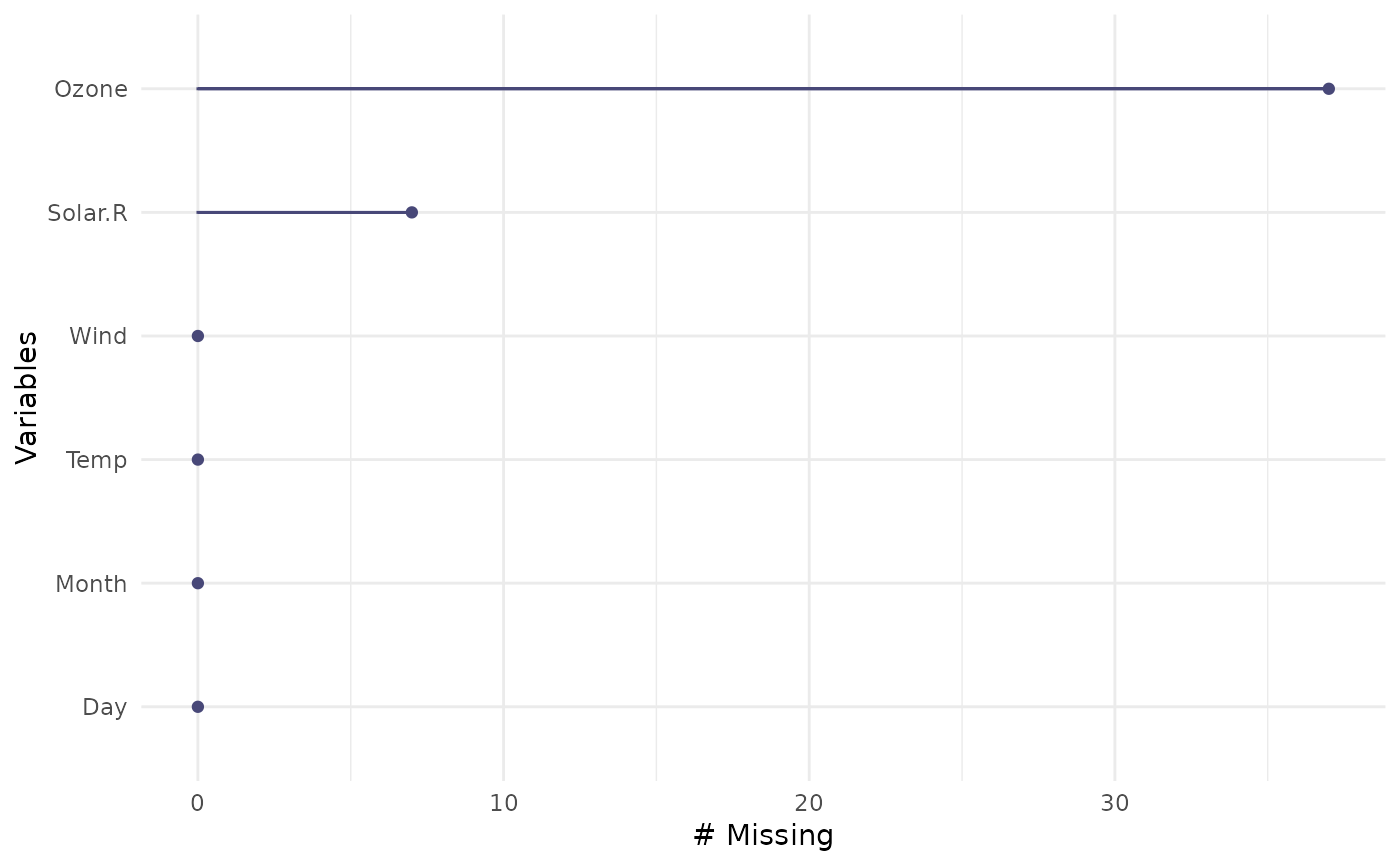This is a visual analogue to miss_var_summary. It draws a ggplot of the number of missings in each variable, ordered to show which variables have the most missing data. A default minimal theme is used, which can be customised as normal for ggplot.

## Usage

gg_miss_var(x, facet, show_pct = FALSE)

## Arguments

x

a dataframe

facet

(optional) bare variable name, if you want to create a faceted plot.

show_pct

logical shows the number of missings (default), but if set to TRUE, it will display the proportion of missings.

## Value

a ggplot object depicting the number of missings in a given column

geom_miss_point() gg_miss_case() gg_miss_case_cumsum gg_miss_fct() gg_miss_span() gg_miss_var() gg_miss_var_cumsum() gg_miss_which()

## Examples


gg_miss_var(airquality)if (FALSE) {
library(ggplot2)
gg_miss_var(airquality) + labs(y = "Look at all the missing ones")
gg_miss_var(airquality, Month)
gg_miss_var(airquality, Month, show_pct = TRUE)
gg_miss_var(airquality, Month, show_pct = TRUE) + ylim(0, 100)
}